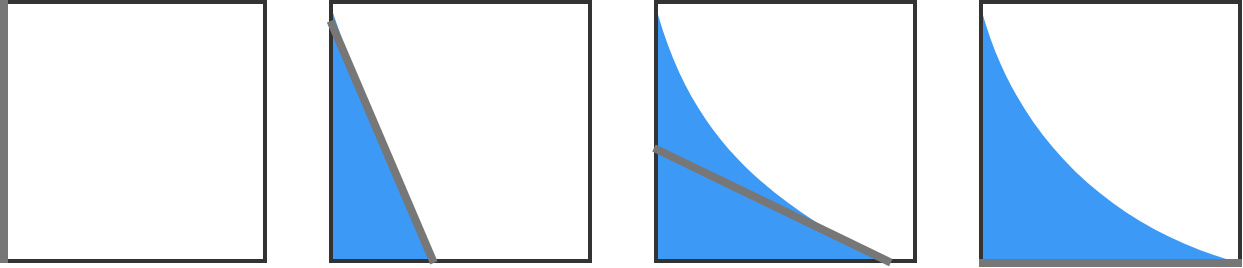# Squeegees

Calculus Level 3

An infinitely thin, $k\text{ cm}$-long squeegee begins to slide down from the upper-left corner of a $k\text{ cm} \times k\text{ cm}$ square window. Its other end simultaneously slides toward the lower-right corner of the window, with the upper end kept in contact with the left side of the window.

If $k=\sqrt{\dfrac{2016}{\pi }}$, what is the area of the window $\big($in $\text{cm}^2\big)$ cleaned by the squeegee?Note: you may end up with an integral expression that's difficult to evaluate analytically. Feel free to finish the job using the code environment below:

import math
Python 3
You need to be connected to run code

×